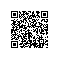# PHP高级编程之消息队列

## PHP高级编程之消息队列

### http://netkiller.github.io/journal/php.mq.html文档出处: http://netkiller.github.io http://netkiller.sourceforge.net2015-10-20

## 3. 什么场合使用消息队列

MQ与RPC有什么不同？ MQ通常传递无规则协议，这个协议由用户定义并且实现存储转发；而RPC通常是专用协议，调用过程返回结果。

1. 存储转发

2. 分布式事务

3. 发布订阅

4. 基于内容的路由

5. 点对点连接

## 5. 谁负责处理消息队列

1. 开发者不用学习消息队列接口
2. 开发者不需要关心消息推送与接收
3. 开发者通过统一的API推送消息
4. 开发者的重点是实现业务逻辑功能

## 6. 怎么实现消息队列框架

https://github.com/netkiller/SOA

### 6.1. 守护进程

https://github.com/netkiller/SOA/blob/master/bin/rabbitmq.php

'Namespace'=>'namespace',
"Class"=>"Email",
"Method"=>"smtp",
"Param" => array(
$mail,$subject, $message, null ) );  序列化后的协议 {"Namespace":"single","Class":"Email","Method":"smtp","Param":["netkiller@msn.com","Hello"," TestHelloWorld",null]}  使用json格式是考虑到通用性，这样推送端可以使用任何语言。如果不考虑兼容，建议使用二进制序列化，例如msgpack效率更好。 ### 6.3. 消息队列处理 消息队列处理核心代码 https://github.com/netkiller/SOA/blob/master/system/rabbitmq.class.php 所以消息的处理在下面一段代码中进行 $this->queue->consume(function($envelope,$queue) {

$speed = microtime(true);$msg = $envelope->getBody();$result = $this->loader($msg);
$queue->ack($envelope->getDeliveryTag()); //手动发送ACK应答

//$this->logging->info(''.$msg.' '.$result)$this->logging->debug('Protocol: '.$msg.' ');$this->logging->debug('Result: '. $result.' ');$this->logging->debug('Time: '. (microtime(true) - $speed) .''); });  public function loader($msg = null) 负责拆解协议，然后载入对应的类文件，传递参数，运行方法，反馈结果。

Time 可以输出程序运行所花费的时间，对于后期优化十分有用。

### 6.4. 测试


<?php
$queueName = 'example';$exchangeName = 'email';
$routeKey = 'email';$mail = $argv;$subject = $argv;$message = empty($argv) ? 'Hello World!' : ' '.$argv;

$connection = new AMQPConnection(array( 'host' => '192.168.4.1', 'port' => '5672', 'vhost' => '/', 'login' => 'guest', 'password' => 'guest' ));$connection->connect() or die("Cannot connect to the broker!\n");

$channel = new AMQPChannel($connection);
$exchange = new AMQPExchange($channel);
$exchange->setName($exchangeName);
$queue = new AMQPQueue($channel);
$queue->setName($queueName);
$queue->setFlags(AMQP_DURABLE);$queue->declareQueue();

$msg = array( 'Namespace'=>'namespace', "Class"=>"Email", "Method"=>"smtp", "Param" => array($mail, $subject,$message, null
)
);

$exchange->publish(json_encode($msg), $routeKey); printf("[x] Sent %s \r\n", json_encode($msg));

\$connection->disconnect();



## 8. 延伸阅读

PHP高级编程之守护进程

PHP高级编程之多线程使用钉钉扫一扫加入圈子
+ 订阅